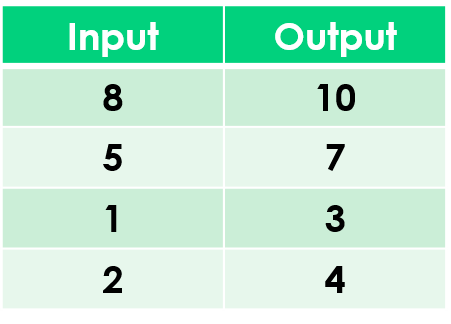1
visibility

Find the rule from the table below:• A

Add 2

• B

Add 3

The correct answer is "add 2".

Please Note:
Addition is the operation that increases a number by adding to it.

Solution:

To find the correct rule, we consider the given table.

We observe that the given rule makes each number increase. This implies addition.

We try adding 2 to each input number:

8 + 2 = 10
5 + 2 = 7
1 + 2 = 3
2 + 2 = 4

On adding 2 to the input numbers, we get the corresponding output numbers.

So, the rule is "add 2”.